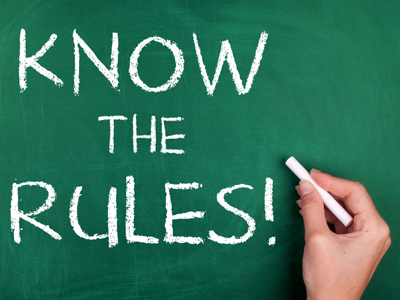We use formulae, or rules, to calculate perimeter and area.

# Perimeter and Area 2 (Medium)

This is the second of our medium level Eleven Plus maths quizzes on perimeter and area. In the first, we looked at ways to work these out with different shapes – the rules for circles are quite different to the rules for rectangles!

• The formula for the area of a square is: Area = length2
• The formula for the area of a right-angle triangle is: Area = 12 × base × height
• The formula for the area of a circle is: Area = πr2
• The formula for the circumference of a circle is: Circumference = 2πr
• π = 227 or 3.142

And now it’s time to put all this to the test. Let’s see if you are a master of perimeter and area.

1.
If the circumference of a circle is 44 cm, what is its radius (assume that π = 3.142 and round your answer to the nearest whole number)?
7 cm
8 cm
9 cm
10 cm
Circumference = 2πr, so 44 = 2 × 3.142 × r
We can rearrange the problem to, r = 44 ÷ (3.142 x 2):
So, r = 44 ÷ 6.284 = 7
2.
If the diameter of a circle doubles, what will happen to the area?
It will quintuple (multiply by 5)
It will quadruple (multiply by 4)
It will treble (multiply by 3)
It will double (multiply by 2)
As you know, diameter is twice radius. In circles, area = πr2. If r = 1 cm, A = 3.142 × 1 × 1 = 3.142 cm. Now, if r = 2, A = 3.142 × 2 × 2 = 12.568.
It’s not obvious at first but 12.568 is four times 3.142. Work it out and see for yourself.
3.
A heptagon is a seven-sided shape. What is the perimeter of a regular heptagon if one of its sides is of length 19.3 cm?
372.49 cm
135.1 cm
115.8 cm
193 cm
A regular heptagon has ALL its sides the same length ∴ perimeter = 7 × 19.3 = 135.1 cm
4.
The area of a wall is 12 m2. A can of paint can cover an area of 5 m2. How many cans of paint will be required to cover the wall?
1
4
2
3
12 ÷ 5 = 2.4. However, you cannot but 0.4 cans of paint – you would have to buy 3. Watch out for questions like this in exams – don’t let them trick you
5.
If you double the length of the sides of a square, what will happen to its area?
It will stay the same
It will double (multiply by 2)
It will treble (multiply by 3)
It will quadruple (multiply by 4)
Suppose we have a square of side length 4 cm. Its area = 4 x 4 = 16 cm2
Now we double the length of its sides. Its area is now 8 x 8 = 64 cm2
64 ÷ 16 = 4. The area has quadrupled in size
6.
A right-angled triangle has a side length of 12 cm. How many of these triangles will fit onto a square whose sides are 28 cm long, without overlapping or going over the edge of the square?
10
5
15
7
The area of square = 28 × 28 = 784 cm2.
Area of the triangles = 12 × base × height = 12 × 12 × 12 = 72 cm2.
The number of triangles = 784 ÷ 72 = 10.888. However, the question says, ‘without overlapping or going over the edge of the square’. This means that remainders are not counted. The answer is 10
7.
The surface area of a cube is 384 cm2. How tall is the cube?
8 cm
16 cm
32 cm
64 cm
A cube has six faces: each face is a square. So, to find the area of each face, divide 384 by 6:
384 ÷ 6 = 64. Next, we want to find the length of each square. The formula for the area of a square is: Area = length2.
If you know your times tables then you should know that 8 x 8 = 64
8.
The area of a right-angled triangle is 225 cm2. If its base is 15 cm, what is its height?
10 cm
20 cm
30 cm
40 cm
Area = (base × height) ÷ 2:
225 = (15 x height) ÷ 2:
2 x 225 ÷ 15 = height:
450 ÷ 15 = height = 30 cm
9.
If the diameter of a circle is 14 cm, what is its circumference (assume that π = 3.142)?
138.21 cm
43.988 cm
87.976 cm
21.994 cm
The circumference of a circle = 2πr. As you know, diameter = 2r so part of this problem was already done for you
10.
The perimeter of a square is 28 cm. What is its area?
784 cm2
196 cm2
49 cm2
7 cm2
The perimeter of a square is 4 x its length. 28 ÷ 4 = 7
The area of a square is length x length. 7 x 7 = 49
Author:  Frank Evans